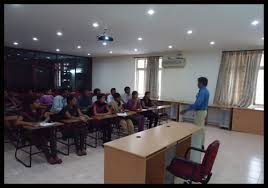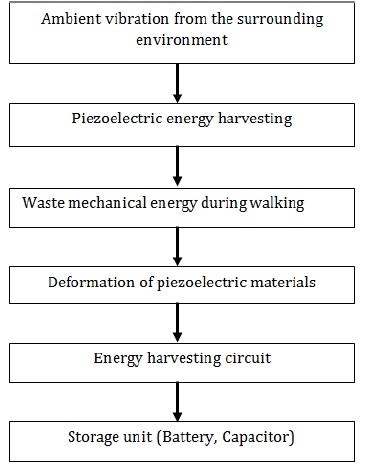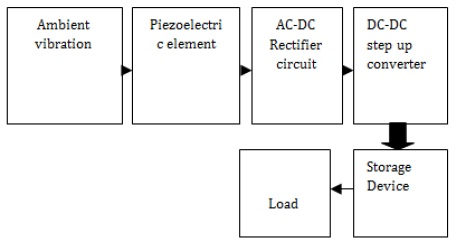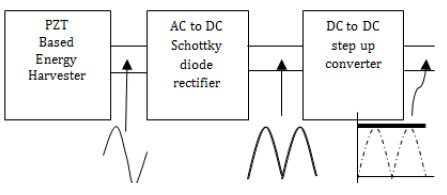## Piezoelectric Energy Harvester

Published on Sep 10, 2023

### Abstract

This paper presents the design of an energy harvesting system using piezoelectric materials for power generation from the shoe sole. A DC-DC step up converter has been chosen for designing of the low extracted energy harvested from the piezoelectric material as PZT. The circuit consumes very little power for its operation. The energy harvesting circuit consists of Schottky diode based ac-dc rectifier, dc-dc converter, capacitor and resistive load at the output terminal in place of rechargeable battery. In this study a simple trigger circuit is used to control switch for synchronizing the DC-DC step up converter circuit.

The simulation results shows that maximum of 1.8mW power is harvested which is sufficient to power low power portable consumer electronic devices like wireless sensors like low power switches and wearable electronics.

### Introduction

Over the last two decade, several articles have reported the use of transduction mechanisms for low power generation from ambient vibrations. As stated by Williams and Yates, there are three basic mechanisms to electric energy conversion that are electromagnetic (P. Glynne-Jones, et al, 2004), electrostatic (P. Mitcheson, et al, 2004) and piezoelectric transductions (S. P. Roundy, et al, 2003). The conversion of mechanical vibration-to-electricity was first investigated and proposed by (C. B. Williams, et al, 1996). Piezoelectric materials have been used to transform ambient mechanical vibrations into electrical energy according to the principle of its (S.P Timoshenko,1953)

.Micro Energy Harvesters are small electromechanical devices, which harvest ambient energy and then convert it into electrical energy. Figure 1 shows a block diagram of piezoelectric ambient vibration energy harvesting system. The choice of the source depends primarily on the specification of the power requirement for an application and design feasibility (K.Finkenzeller, 2003). The process of extracting unused energy from the ambient environment and converting it into a usable form of electrical energy is known as Energy Harvesting.

With recent growth in the development of low-power electronic devices such as microelectronics and wireless sensor nodes, piezoelectric materials has received the most attention for obtaining electric energy from the surrounding environment for their ability to directly convert vibrations into electrical energy. Rectified electrical energy harvested from piezoelectric material is very low to operate low-power electronic devices, so an interface circuit is required, for this many studies have been devoted in the literature to optimize the interfacing circuit to maximize the power output of a piezoelectric transducer on various resistive loads.For example, dc–dc converter circuits have been implemented to match the actual load to the impedance of the piezoelectric transducer, thus maximizing the power transfer (G. Ottman, et al, 2002). Comparison of one-stage and two-stage energy harvesting schemes were studied and investigated. The results show that one-stage energy harvesting scheme can achieve higher efficiency than the two-stage scheme as DC–DC converter consume a certain part of collected energy (M J Guan,et al,2007). The paper is also organized as follows: Section II describes the modeling of piezoelectric based Energy Harvesting system, such as piezoelectric element, AC-DC rectifier, temporary storage devices, DC-DC Converter

### Development of piezoelectric energy harvesting circuit

This section gives the details related to modeling of piezoelectric energy harvesting system consisting of piezoelectric element, AC-DC rectifier, DC-DC step up converter, capacitor and resistive load. Figure 2 shows the block diagram representation of the circuit with the details of each block. For the development of energy harvesting system, the TINA software has been used to implement the proposed system.### Piezoelectric element

The piezo term comes from a Greek word piezein for pressure. Most common type of piezoelectric material used in energy harvesting applications is lead zircon ate titan ate, piezoelectric ceramic, or piezo ceramic also known as PZT. When pressure is applied on PZT, it converts the applied pressure into electrical potential difference, which is irregular function of time (i.e. AC signal) and having very less amplitude. For converting AC signals to stable DC, an AC to DC full wave rectifier is used.

### AC-DC rectifier circuit

The efficiency of full wave bridge rectifier circuit is very low i.e. the forward voltage drop of diode is higher than the applied input signals. To eliminate this problem, Schottky barrier diodes are used to get suitable DC voltage. Normal diodes have forward voltage drop of 0.7 volts and Schottky barrier diodes have forward voltage drop of 0.3 volts. In our research, we have used Schottky barrier diodes to design AC-DC rectifier circuit. The rectified output is applied to DC-DC step up converter, which provides the higher output than applied input. Figure 3 shows the proposed block diagram of the AC-DC rectifier.

### DC-DC step-up converter

The third block of the energy harvesting circuit is the DC-DC step-up converter and its schematic diagram shown in Figure 4.The corresponding block diagram is shown in Figure 2 and results shown in section 3.The development of DC-DC converter provides a boost-up output while input voltage is too low. The development of DC-DC converter used the DC input (Vin) = 0.133V.The switch (T1) remains in open state till the maximum mechanical pressure is applied on the piezoelectric element. At that position, the switch is turn to close position and the capacitance of the piezoelectric element and inductor together constitute an oscillator working on resonance frequency. The switch is kept closed until the voltage on the piezoelectric element has been reversed.

Very high energy harvesting capacity at the output can be obtained from this circuit arrangement. The advantage of new developed DC-DC boost converter is that it is able to increase the DC voltage using very low input source. We used (T1) transistor and trigger pulse (Tp) is V1=0V, V2=0.8V TD=500us, TR= 8m, TF=500us, PW=8ms as a switching purpose for open and close of switch. If a large capacitor (C1) is connected across the load as the output voltage is continuous and Vout becomes the average value that the voltage across the load can be stepped up by varying the duty cycle and the minimum output voltage is Vin when duty cycle = 0. We used inductor (L1) of 100mH for storage the current, Resistor (R1) is 100kΩ used for the better output, we used capacitor (C1) of 10uF for storing voltage and providing filtering actions. Finally we achieve the output voltage Vout of 4.29 V after using the DC-DC converter (Step-up).

### CONCLUSIONS

The energy harvesting circuits consisting of piezoelectric element, AC-DC rectifier, , DC-DC converter (Step-Up) and temporary storage device have developed by TINA PRO simulation. The main focus of the research work is to develop the DC-DC Step-Up Converter which achieves the maximum output voltage of 4.29V. Simulation results shows that maximum of 1.8mW of power is harvested from DC-DC step up converter, which can be sufficient to power low power portable devices such as wireless sensor such as low power switches and consumer electronics. Future work will be focused on the design of the hardware implementation to verify the simulation result etc.

### REFERENCES

S.P Timoshenko,(1953),History of Strength of Materials (with a brief account of the history of theory of elasticity and theory of structures) New York: McGraw-Hill Book Company.

C. B. Williams and R. B. Yates, (1996), Analysis of a Micro-electric Generator for Microsystems, Sensors and Actuators A, pp. 8–11.

P. Glynne-Jones, M. J. Tudor, S. P. Beeby, and N. M. White,(2004) An Electromagnetic, Vibration-powered Generator for Intelligent Sensor Systems, Sensors and Actuators A, 110, pp. 344-349.

P. Mitcheson, P. Miao, B. Start, E. Yeatman, A. Holmes and T. Green, (2004),MEMS Electrostatic Micro-Power Generator for Low Frequency Operation, Sensors and Actuators A, 115, pp. 523-529.

S. P. Roundy, P. K. Wright, and J. M. Rabae,(2003),A Study of Low Level Vibrations as a Power Source for Wireless Sonsor Nodes, Computer Communications, pp.1131-1144.

 Are you interested in this topic.Then mail to us immediately to get the full report. email :- contactv2@gmail.com